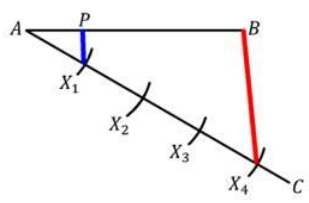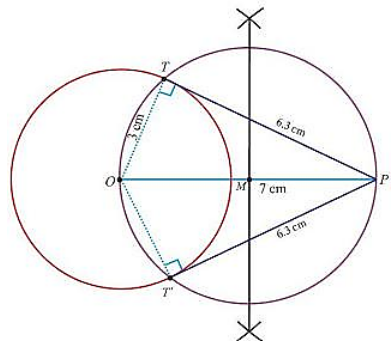×#### Thank you for registering.

One of our academic counsellors will contact you within 1 working day.

Click to Chat

1800-1023-196

+91-120-4616500

CART 0

• 0

MY CART (5)

Use Coupon: CART20 and get 20% off on all online Study Material

ITEM
DETAILS
MRP
DISCOUNT
FINAL PRICE
Total Price: Rs.

There are no items in this cart.
Continue Shopping• Complete JEE Main/Advanced Course and Test Series
• OFFERED PRICE: Rs. 15,900
• View Details

```Revision Notes on Constructions

Division of a Line Segment

If we have to divide a line segment in particular ratio, then we can do it by measuring the length on the ruler and mark it on the line. But if we don’t have anything to measure then we can do it by using steps of construction.

Method 1AB is a line segment of 4 cm. Divide it in the ratio of 1:3 using a compass.

Steps of Construction

Step 1: Draw a line AC of any length by making an acute angle with the given line segment AB.

Step 2: Using any small length on the compass mark 4 points of equal size on AC, so that AX1 = AX2 = AX3 = AX4.We are marking 4 points as we have to divide the line in the ratio of 1:3, so 1 + 3 = 4.

Step 3: Now join BX4.

Step 4: Draw a line from point X1 to line AB parallel to BX4, which intersects AB at point P.

Now AP: PB = 1:3.Method 2(Alternative Method)

A line can also be divided by another method.

Steps of Construction

Step 1: Draw a line AC with the acute angle with the line segment AB.

Step 2: Draw another line DB parallel to AC so that ∠BAX = ∠ADB

Step 3: Mark the points X1(m = 1) on AC and Y1, Y2, Y3 n = 3) on DB so that AX1 = BY1 = Y1Y2 = Y2Y3.

Step 4: Join X1Y3 so that it intersects line AB at P.

AP:PB = 1:3

Construction of a Triangle similar to a given Triangle as per given Scale

The scale factor is the ratio of the sides of the triangle given to the sides of the triangle to be made by the steps of construction.Example:

Draw a triangle similar to ∆ABC with its sides equal to 2/3 of the corresponding sides of the given triangle ABC. (Scale factor = 2/3).

Steps of Construction

Step 1: Draw a line AX by making an acute angle with the line segment AB.

Step 2: Mark three points of equal size using a compass on the line AX. Points will be depending upon the scale factor as we have to mark the number of points which is greater in the scale factor. In the ratio of 2/3(3 > 2).

Step 3: Join BX3 and draw a line from X2 parallel to BX3 to intersect AB at P.

Step 4: Draw a line parallel to BC from point P to intersect AC at Q.

Now ∆APQ ~ ∆ABC.

Remark: Here we have made a similar triangle which is smaller than the given triangle because the scale factor was 2/3. But if we have scale factor like 5/3 then we will make a bigger triangle then the given triangle by taking 5 points on the line).

Construction of Tangents to a Circle

Tangent is a line which intersects the circle at one point only at the outer of the circle. It is always perpendicular to the radius of the circle.

Example:

Construct the pair of tangents to the circle of radius 3 cm from the point which is 7 cm away from its centre, and measure their lengths also.

Steps of Construction

Step 1: Draw a circle of radius 3 cm by taking O as the centre.

Step 2: Mark a point P outside the circle at a distance of 7 cm from the centre O. Join OP.

Step 3: Bisect the line segment OP, so that the perpendicular bisector of OP intersects it at the point M.

Step 4: Now draw another circle by taking M as centre and MO as radius, which intersects the given circle at two points’ i.e. T and Tꞌ.

Step 5: Now join PT and PTꞌ which are the required tangents and measure the length of the tangents.The length of the tangents is PT = PTꞌ = 6.3 cm.
```### Course Features

• 728 Video Lectures
• Revision Notes
• Previous Year Papers
• Mind Map
• Study Planner
• NCERT Solutions
• Discussion Forum
• Test paper with Video Solution